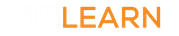### Physics Virtual Lessons

### Intro topic (maths skills)

• Standard Form
• Manipulating formulae

### Energy

• Energy changes in mechanical processes
• Power
• Useful and wasteful energy transfers
• Reducing unwanted energy transfers
• Efficiency
• Energy sources

### Electricity

• Electric Charge and Current
• Voltage and Potential Difference
• Resistance
• Types of Resistor
• Investigating Resistance
• Series Circuits
• Parallel Circuits
• Investigating parallel and series circuits
• Domestic electricity supplies
• Electrical power
• Electrical energy transfers
• The National Grid
• Static electricity
• Electric fields

### Particle model of matter

• Energy and changes of state
• Specific heat capacity
• Gas pressure
• Fluid pressure

### Nuclear Physics

• Atoms and isotopes
• Models of the atom
• Nuclear calculations
• Half-life
• Fission
• Fusion

### Motion

• Speed
• Acceleration
• Distance time graph
• Velocity time graphs
• Stopping distances
• Reaction time
• Braking distance

### Forces

• Newton's second law
• Newton's third law
• Resultant forces
• Gravity
• Forces doing work
• Forces and elasticity
• Rotational forces
• Momentum
• Changes in momentum

### Waves and the electromagnetic spectrum topic

• Describing waves
• Measuring waves
• Sound & hearing
• Waves for detection and exploration
• The electromagnetic spectrum
• Using electromagnetic waves
• Creating electromagnetic waves
• Reflection of waves
• Refraction of waves
• Wave transmission & absorption
• Lenses 1
• Lenses 2
• Colour and visible light

### Magnets

• Magnetic fields
• Electromagnetism
• Electric motors
• The Generator Effect
• Transformers

### Astronomy & Space

• The Life Cycle of Stars
• Gravity and Orbits
• Red Shift and Theories of the Universe